Fit Analyses

## Nonparametric Smoothing Spline

Two criteria can be used to select an estimator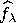for the function f:

• goodness of fit to the data
• smoothness of the fit

A standard measure of goodness of fit is the mean residual sum of squares
A measure of the smoothness of a fit is the integrated squared second derivative
A single criterion that combines the two criteria is then given by
wherebelongs to the set of all continuously differentiable functions with square integrable second derivatives, and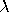is a positive constant.

The estimator that results from minimizing S()is called the smoothing spline estimator. This estimator fits a cubic polynomial in each interval between points. At each point xi, the curve and its first two derivatives are continuous (Reinsch 1967).

The smoothing parametercontrols the amount of smoothing; that is, it controls the trade-off between the goodness of fit to the data and the smoothness of the fit. You select a smoothing parameterby specifying a constant c in the formula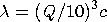where Q is the interquartile range of the explanatory variable. This formulation makes c independent of the units of X.

After choosing Curves:Spline, you specify a smoothing parameter selection method in the Spline Fit dialog.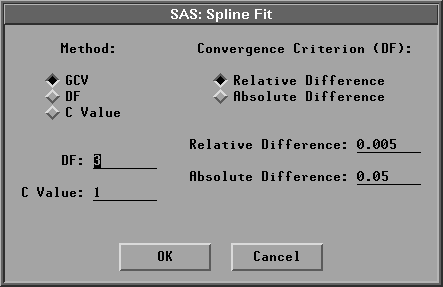Figure 39.40: Spline Fit Dialog

The default Method:GCV uses a c value that minimizes the generalized cross validation mean squared error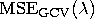.Figure 39.41 displays smoothing spline estimates with c values of 0.0017 (the GCV value) and 15.2219 (DF=3). Use the slider in the table to change the c value of the spline fit.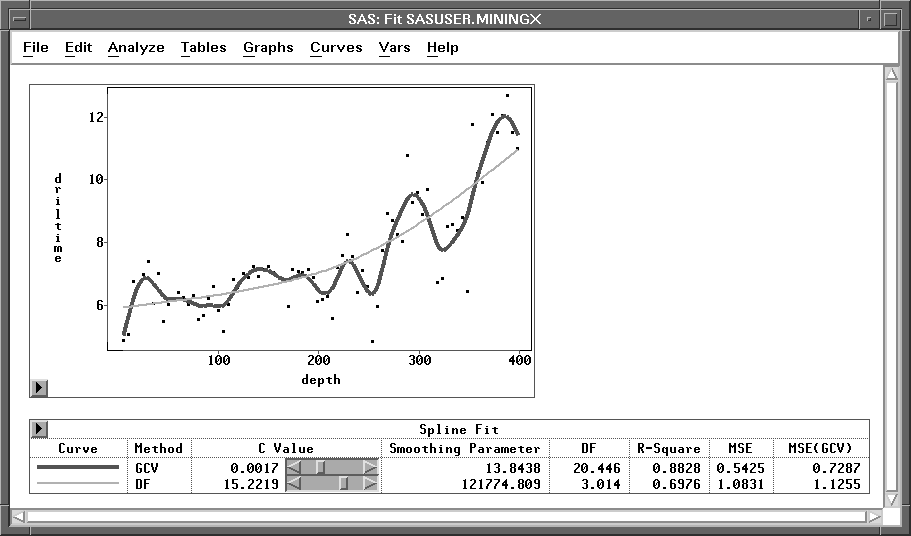Figure 39.41: Smoothing Spline Estimates

Copyright © 2007 by SAS Institute Inc., Cary, NC, USA. All rights reserved.